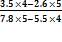```1. 1615 ÷ (50 × 0.85) = ?

(a) 36

(b) 38

(c) 40

(d) 42

(e) None of these

2. 126315 – 87924 = ? × 67

(a) 684

(b) 661

(c) 573

(d) 562

(e) None of these

3. 547 × 37 – 20000 = ?

(a) 239

(b) 237

(c) 235

(d) 233

(e) None of these

4. If (150)2 is added to the cube of a number, the answer so obtained is 77372. What is the number?

(a) 32

(b) 34

(c) 36

(d) 38

(e) None of these

5. 456 ÷ 24 + 324 × 17 = ?

(a) 5525

(b) 5725

(c) 5727

(d) 5427

(e) None of these

6. 5 3/5 × 2  6/7  = ?

(a) 13

(b) 17

(c) 21

(d) 25

(e) None of these

7. √(?) + 18 = √1444

(a) 441

(b) 361

(c) 400

(d) 484

(e) None of these

8. If (51)2 is added to the square of a number, the answer so obtained is 15826. What is the number?

(a) 115

(b) 114

(c) 116

(d) 113

(e) None of these

9. 3412 + 4367 + 5590 = ?

(a) 13121

(b) 13245

(c) 13369

(d) 13487

(e) None of these

10. 23 8/9 + 15  7/9  - 12  1/3  ×  2/3  = ?

(a) 30  1/3

(b) 31  1/3

(c) 30  4/9

(d) 31  4/9

(e) None of these

11.(a) 34

(b) 28

(c) 17

(d) 51

(e) None of these

12. 33.03 × 5.5 + 18.95 = ?

(a) 199.665

(b) 200.605

(c) 200.615

(d) 200.665

(e) None of these

13. 780 ÷ √676 + (?)2 = 1326

(a) 1296

(b) 32

(c) 1024

(d) 36

(e) None of these

14. 1/? × 2/7  ×  4/5  × 4585 = 65.5

(a) 8

(b) 24

(c) 4

(d) 16

(e) None of these

15. 744 ÷ ? × 0.4 = 9.3

(a) 32

(b) 35

(c) 36

(d) 28

(e) None of these

16. 2/3rd of 3/5th of 4/5th of ? = 1112

(a) 3575

(b) 3475

(c) 3425

(d) 3215

(e) None of these

17. If the square of a number is subtracted from 4052 and the difference is multiplied by 15, the answer so obtained is 41340. What is the number?

(a) 36

(b) 1024

(c) 32

(d) 1296

(e) None of these

18. (7)3 + (5)2 + (4)3 ÷ (16)2 = ?

(a) 1.6875

(b) 468.25

(c) 368.0625

(d) 368.25

(e) None of these

19. 4580 – 36% of ? = 4529.6

(a) 180

(b) 120

(c) 150

(d) 140

(e) None of these

20. If (11)3 is subtracted from the square of a number, the answer so obtained is 7694. What is the number?

(a) 85

(b) 93

(c) 95

(d) 83

(e) None of these